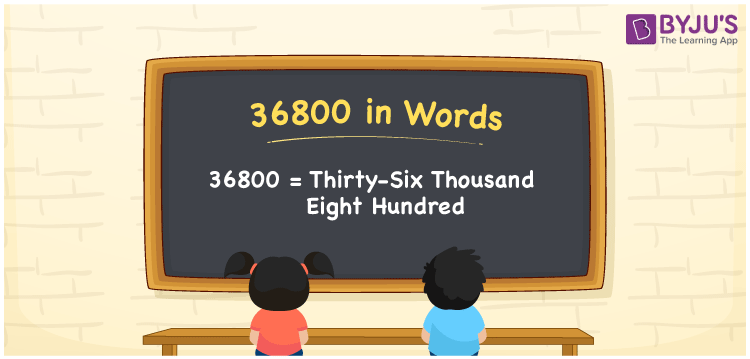# 36800 in Words

36800 in words is thirty-six thousand eight hundred. The conversion from numbers to words becomes easy, if you know the place values of the number. Also, the number 36800 is a cardinal number as it expresses the value. For example, there are 36800 books in a library.

 36800 in Words: Thirty-six Thousand Eight Hundred. Thirty-six Thousand Eight Hundred in Numerical Form: 36800.

## 36800 in English Words## How to Write 36800 in Words?

Learn the place values of 36800 using the below table.

 Ten-thousands Thousands Hundreds Tens Ones 3 6 8 0 0

The expanded form of 36800 is as follows:

= 3 × Ten thousand + 6 × Thousand + 8 × Hundred + 0 × Ten + 0 × One

= 3 × 10000 + 6 × 1000 + 8 × 100 + 0 × 10 + 0 × 1

= 30000 + 6000 + 800

= 36800

= Thirty-six thousand eight hundred

Hence, 36800 in words is thirty-six thousand eight hundred.

36800 in words – Thirty-six thousand eight hundred

Is 36800 an odd number? – No

Is 36800 an even number? – Yes

Is 36800 a perfect square number? – No

Is 36800 a perfect cube number? – No

Is 36800 a prime number? – No

Is 36800 a composite number? – Yes

## Frequently Asked Questions on 36800 in Words

Q1

### Write 36800 in English words.

36800 in words is thirty-six thousand eight hundred.

Q2

### Simplify 36000 + 800, and express it in words.

Simplifying 36000 + 800, we get 36800. Hence, 36800 in words is thirty-six thousand eight hundred.

Q3

### Is 36800 an even number?

Yes, 36800 is an even number.